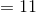# Common Core: 1st Grade Math : Use Properties of Operations to Add and Subtract: CCSS.MATH.CONTENT.1.OA.B.3

## Example Questions

### Example Question #31 : Common Core Math: Grade 1

If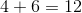, then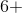__________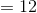Explanation:

This is an example of commutative property. Commutative property of addition means that you can change the order of the smallest two numbers when adding, and it will always equal the biggest number.

### Example Question #32 : Common Core Math: Grade 1

If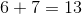, then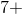__________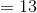Explanation:

This is an example of commutative property. Commutative property of addition means that you can change the order of the smallest two numbers when adding, and it will always equal the biggest number.

### Example Question #31 : Common Core Math: Grade 1

If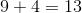, then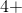__________Explanation:

This is an example of commutative property. Commutative property of addition means that you can change the order of the smallest two numbers when adding, and it will always equal the biggest number.

### Example Question #31 : Common Core Math: Grade 1

If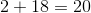, then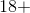__________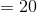Explanation:

This is an example of commutative property. Commutative property of addition means that you can change the order of the smallest two numbers when adding, and it will always equal the biggest number.

### Example Question #31 : Common Core Math: Grade 1

If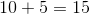, then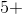__________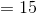Explanation:

This is an example of commutative property. Commutative property of addition means that you can change the order of the smallest two numbers when adding, and it will always equal the biggest number.

### Example Question #32 : Common Core Math: Grade 1

If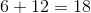, then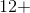__________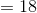Explanation:

This is an example of commutative property. Commutative property of addition means that you can change the order of the smallest two numbers when adding, and it will always equal the biggest number.

### Example Question #37 : Common Core Math: Grade 1

If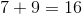, then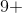__________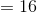Explanation:

This is an example of commutative property. Commutative property of addition means that you can change the order of the smallest two numbers when adding, and it will always equal the biggest number.

### Example Question #32 : Common Core Math: Grade 1

If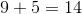, then__________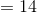Explanation:

This is an example of commutative property. Commutative property of addition means that you can change the order of the smallest two numbers when adding, and it will always equal the biggest number

### Example Question #39 : Common Core Math: Grade 1

If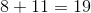, then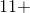__________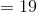Explanation:

This is an example of commutative property. Commutative property of addition means that you can change the order of the smallest two numbers when adding, and it will always equal the biggest number.

### Example Question #40 : Common Core Math: Grade 1

If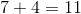, then__________